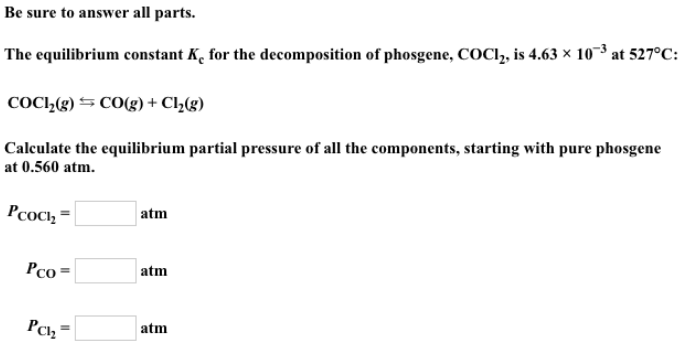# Problem: Be sure to answer all parts.The equilibrium constant Kc for the decomposition of phosgene, COCI2, is 4.63 x 10-3 at 527 °C: COCI2 (g) ⇋ CO (g) + CI2 (g) Calculate the equilibrium partial pressure of all the components, starting with pure phosgene at 0.560 atm. PCOCI2 = PCO = PCI2 =

###### FREE Expert Solution
85% (2 ratings)###### Problem Details

Be sure to answer all parts.

The equilibrium constant Kc for the decomposition of phosgene, COCI2, is 4.63 x 10-3 at 527 °C:

COCI2 (g) ⇋ CO (g) + CI2 (g)

Calculate the equilibrium partial pressure of all the components, starting with pure phosgene at 0.560 atm.

PCOCI2

PCO =

PCI2 =